## 2.2.3 Carrier Continuity Equations

The space charge density in semiconductors is composed of the charges of electrons, holes, and the ionized dopant atoms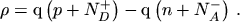(2.37)

POISSON's equation (2.14) can be rewritten as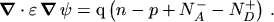(2.38)

Since the current relations were introduced by using two separate subsystems it is convenient to also split up the current continuity equation eqn. (2.18) into two equations by introducing a formal separation parameter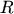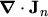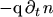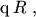(2.39)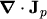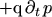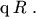(2.40)can be interpreted as the net recombination rate defined as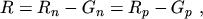(2.41)

where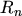/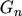and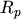/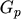are the recombination/generation rates for electrons and holes, respectively.

By summarizing POISSON's equation (2.38), the current continuity equations (2.39) and (2.40), and the current relations (2.35) and (2.36), the set of basic semiconductor equations is obtained. This set of equations was first presented by VANROOSBROECK in 1950 .

M. Gritsch: Numerical Modeling of Silicon-on-Insulator MOSFETs PDF